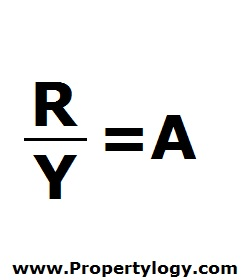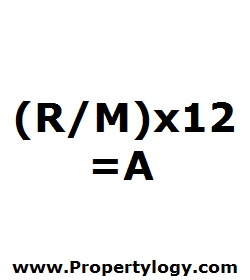Formula - Annualized Return | Propertylogy
Don't Miss

Formula – Annualized Returns

By on June 10, 2018

There are 2 ways that annualized returns are often presented.

1. Years
2. Months

Here’s both of them.R/Y = A

R – Return over holding period

Y – Holding period in years

A – Annualized returns (Years)(R/M) x 12 = A

R – Return over holding period

M – Holding period in months

A – Annualized returns (Months)

When it is stated that an investment is achieving a certain amount of returns, it is important to put the time frame into perspective.

It might sound impressive if an asset is declared to be yielding 20% returns. But it would look totally different if it is an investment over 20 years.

Because the standard industry practice is to calculate returns on investment on a yearly basis, the annualized return equations enable an analysis to determine the annual returns of a long running investment that has extended for years.

Send this to a friend